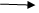Break and continue Statements in C
Ratings:
(4)
Views:534## About Break and continue Statements in C LanguageBreak is a keyword, by using break we can terminate loop body or switch body.Using break is always optional but it must be placed within loop body or switch body only.In implementation where we know the maximum number of repeat ions but depends on the condition if we required to stop the repeat ion then use break statement.Continue is a keyword; by using continue we can skip statements from the loop body.Using continue is always optional but it must be placed within the loop body only.In implementation where we knows the maximum number of reparation but depends on the condition if we required to skip the statements from loop body then go for continue.

• ### Void main()

{ Inta; A=1; While(a<=10) { Printf(“%d”,a); If(a>3) Break; ++a; } } O/p : 1234 a=1; while (a<=10) { pf(“%d”,a); If(a>3) Break; ++a;

• ### Void main()

{ Inta; A=2; While(a<=20) { Pf(“%d”,a); a+=2; if(a>=8) break; } } O/p: 246

• ### Void main()

{ Int a; A=15; While(a>=1) { A-=2; If (a<9) Break; Pf(“%d”,a); } } O/p: 13   11  9

• ### Void main()

{ Int a; A=1; While(a<=10) { Pr(“%d”,a); If(!a) Break; A+=2; } } O/p:  1 3 5 7 9

• ### Void main()

{ int a; A=5; While(a<=50) { pf(“%d”,a); If(a>=25)  dummy condition Break; A+=5; } } O/P: 5 While(a<=50){ Pf(“%d”,a); If(a>=25) { } Break; A+=5; }

• ### Void main()

{ int a; A=1; While (a<=50) dummy loop { Printf(“%d”,a); If(a>=25) Break; A+2; } } O/p : Error misplaced break. While(a<=50) { } { Pf(”%d”,a); If (a>25) Break; A+=2; }When we are constructing the dummy loop then complier will create new body without any statement and current body became outside o f the loop.In above program duet to dummy loop break statement is placing outside the body so it is an error (we cannot palace outside)

• ### Void main()

{ Int a; A=0; While(a<=100) { A+=2; If (a>=40&&a<=80) Continue; Printf(“%d”,a); } O/p: 2 4 6  . . . . .  .38 82 84 86 …….98 100 102.When continue statement is executing then control will pass back to the condition without executing remaining statement with in the body.

• ### Void main()

{ Int a; A=1; While(a<75) { A+=2; If (a.25 && a<55) Continue; Printf(“%d”,a); } } O/p:  3 5 7 . .  . . .  . . .  . . .23 25  55 57 59 ….. 73 75

• ### Void main()

{ Int a; A=60; While(a>=2) { Printf(“%d”,a); If(a>=20 && a<=50) Continue; A=-2; } } O/p: 60 58 56 54 52 50 50 50 50 ……….. infite loop cntrl break.

• ### Void main()

{ Int a; A=1; While(a<=200) { if (a>=5 &&a<=150) continue ; printf(“%d”,a); ++a; } } O/p: 1 2 3 4 no output with infinite loop  (cntrl break)

• ### Void main()

{ Int a; a>=100; while(a.=10); { a-=2; if(a>=30 && a<=10) continue; printf(“%d”,a); } } O/p : error misplaced continue.